# Chapter 3 - AC Machine - Notes, Electrical Machines, Electrical Engineering Notes - Electrical Engineering (EE)

## Electrical Engineering (EE): Chapter 3 - AC Machine - Notes, Electrical Machines, Electrical Engineering Notes - Electrical Engineering (EE)

The document Chapter 3 - AC Machine - Notes, Electrical Machines, Electrical Engineering Notes - Electrical Engineering (EE) is a part of Electrical Engineering (EE) category.
All you need of Electrical Engineering (EE) at this link: Electrical Engineering (EE)

# The transformer is said to be loaded, when its secondary circuit is completed through an impedance or load. The magnitude and phase of secondary current (i.e. current flowing through secondary) I2 with respect to secondary terminals depends on the characteristic of the load I.e. Current I2 will be in phase., lag behind and lead the terminal voltage, V2 respectively when the load is non- inductive, inductive and capacitive. The net flux passing through the core remains almost constant from no load to full load irrespective of load conditions and so core losses remain almost constant from no load to full load.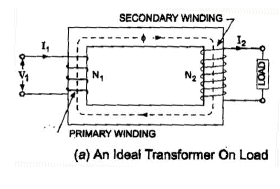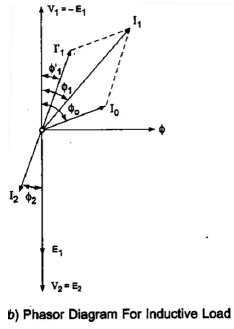Resistance and Leakage Reactance.

In actual practice, both of the primary and secondary windings have got some ohmic resistance causing voltage drops and copper losses in the windings.

In actual practice, the total flux created does not link both of the primary and secondary windings but is divided into three components namely the main or mutual flux f linking both of the primary and secondary windings, primary leakage flux fL1 linking with primary winding only and secondary leakage flux fL2 linking with secondary winding only. The primary leakage flux fL1 is produced by primary ampere-turns and is Proportional to primary current, number of primary turns being fixed. The Primary leakage flux fL1 is in phase with I1 and produces self induced emf ELI given as 2pfL1 I1 in the primary winding. The self induced emf divided by the primary current gives the reactance of primary and is denoted by X1.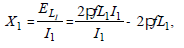Similarly leakage reactance of secondary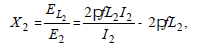When a practical transformer is loaded Induced emf in secondary winding

E2 = V2 + I2 (R2 + j X2) = V2 + I2 Z2

Induced emf in primary
E1 = E2/k

and applied voltage to primary winding.

V1 =E1 + I1 (R1 + jX1) =–E1 + I1 Z1

Equivalent Resistance and Reactance.

The equivalent resistances and reactance of transformer referred to primary and secondary sides are given as follows.
Equivalent resistance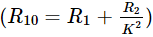Equivalent reactance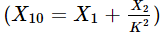Referred to secondary side Equivalent resistance R02 =K2 R1 +R2

Equivalent reactance X02 =K2 X1 + X2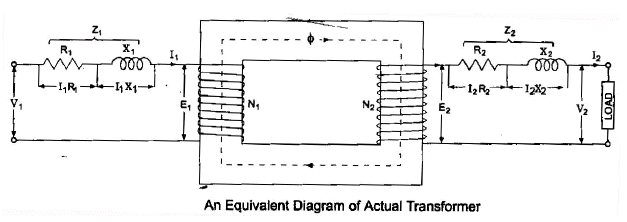Equivalent Circuit

The equivalent circuit of any device can be quite helpful in predetermination of the behaviour of the device under various condition of operation and it can be drawn if the equation describing its behaviour are known. Equivalent circuit of a transformer having transformation ratio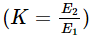is shown in fig.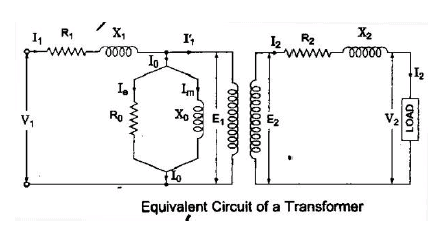Voltage Regulation

The figure of merit which determines the voltage characteristic of a transformer is termed as voltage regulation.
It is defined as the change in magnitude of terminal (secondary) voltage when full-load (rated load) of specified power factor supplied at rated voltage is thrown off (reduced to no load) with primary voltage and frequency held constant, as percentage of the rated terminal voltage. The percentage regulation can be given as.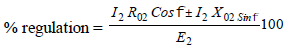+ ve sing for lagging power factor and – ve sign for leading power factor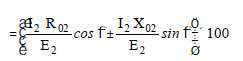= (PU resistance × cos f± PU reactance sin f) × 100

Voltage regulation of a transformer, on an average, is about 4 per cent. Regulation will be zero when power factor angle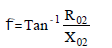The-ve sign indicates that zero regulation occurs at a leading power factor. Regulation will be maximum when power factor angle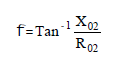Maximum regulation occurs at lagging power factor.

Transformer losses

Since the transformer is a static machine, so there are no friction and windage losses, Hence the losses occurring in a transformer are (i) iron loss and (ii) copper loss. Iron loss is caused by the alternating flux in the core and consists of hysteresis and eddy currents. The copper loss occurs due to ohmic resistance of the transformer windings. The iron or core loss remain almost constant, where as copper loss varies as the square of the load current. For example if copper loss at full load is Pc, then at one-half of the full load the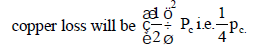Transformer Tests

Open circuit or No-load Test This test is performed to determine core iron loss Pi and no-load current I0. This test is heopful in determination of magnetizing component Ie and so noload resistance R0 being given as V1/ Ie and no- load reactance given as V1/Im.

In this test secondary (usually high voltage ) winding is left open, all metering instruments (ammeter, voltmeter and wattmeter) are connected on primary side and normal rated voltage is applied to the primary (low voltage) winding, as illustrated in fig.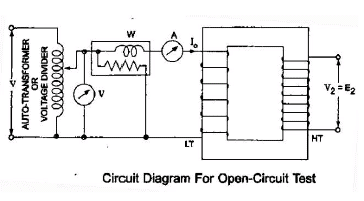The open- circuit test gives enough data to compute the equivalent circuit constants R0, X0, no-load power factor cos f0, no- load current I0 and no- load power loss (iron loss) of a transformer. Iron loss, Pi = Input power on no load = P0 watts (say)

No- load current = I0 amperes
Applied voltage to primary = V1 volts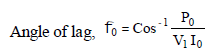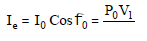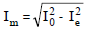Equivalent circuit parameter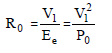Equivalent circuit Parameter,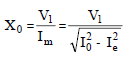Since no- load current I0 is very small, therefore, pressure coils of wattmeter and the voltmeter should be connected such that the current taken by them should no flow through the current coil of the wattmeter.

2. Short- circuit or impedance Test

This test is performed to determine the full- load copper loss and equivalent resistance and reactance referred to secondary side. In this test, the terminals of the secondary (usually the low-voltage) winding are short circuited, all meters (ammeter, voltmeter and wattmeter) are connected on primary side and a low voltage, usually 5 to 10% of normal rated primary voltage at normal frequency is applied to the primary, as shown in Fig. The applied voltage to the primary, say, Vs is gradually increased till the ammeter A indicates the full- load current of the side in which it is connected. The reading Ws of the wattmeter gives total copper loss (iron losses being negligible due to very low applied voltage resulting in very small flux linking with the core) at full load. Let the ammeter reading be Is.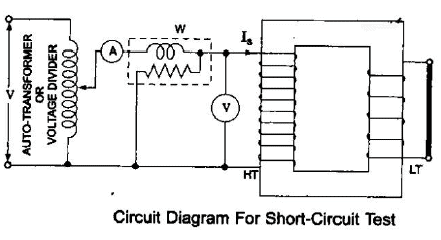Full-load copper oss,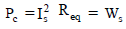Equivalent resistance,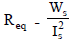Equivalent Impedance,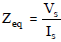Equivalent reactance,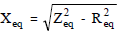3. Sumpner’s Test

Sumpner’s test ,also called the back to back test, is the most important test for determination of rise of maximum temperature of a transformer. For this test two identical transformers are requited which ate loaded fully in this test but the power required from the supply is that necessary for supplying the iron and copper losses of both of the transformers.

Commercial Efficiency

Commercial Efficiency is defined as the ratio of power output to power input in killowatts and is given as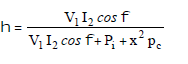where x is the ratio of secondary current I2 and rated full- load secondary current. Transformer efficiency will be maximum when variable losses (copper losses ) are equal to constant losses (iron or core losses) and output k VA corresponding to maximum efficiency.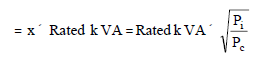All- day or Energy Efficiency

The performance of such a transformer must be judged by its all day efficiency, also called the energy efficiency or operational efficiency which computed on the basis of energy consumed during the while day (24 Hours)
The all- day efficiency is defined as the ratio of energy (kWh) output over 24 hours to the energy input over the same period.

(i.e All-day efficiency =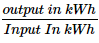Since the distribution transformer does not supply the rated load for the whole day so the all-day efficiency of such a transformer will be less than ordinary or commercial efficiency.

Transformer Construction

The transformer is very simple in construction and consists of magnetic circuit linking with two windings known as primary and secondary windings.

Besides magnetic circuit and windings it consists of a suitable container for the assembled core and windings, such as a tank, a suitable medium for insulating the core and windings from its container such as transformer oil, suitable bushings (either of porcelain, Oil-filled or condenser type) for insulation ad bringing out erminals of the windings from the container, temperature gauge for measurement of temperature of hot oil or hottest spot temperature, and oil gauge to indicate the oil level inside the tank. Some transformer are also provided with conservator tank in order to slow down deterioration of oil. Magnetic circuit consists of an iron core.

Ideally the steel, of which the transformer core is made, is simple in chemical composition, the hot rolled steel has 4-5% silicon and the cold rolled has 3% .

Since flux is an alternating one, in order to reduce eddy current losses the core is laminated. The thickness of lamination or stampings varies from 0.35 mm to 0.5 mm. The laminations are insulated from each other by coating them with a thin coat of varnish. The iron core is rectangular in x-section for small size transformers and circular for large size ones to reduce the quantity of copper required.

Type of Transformers

Depending upon the type of construction used, the transformers are classified into two categories viz. (i) core type and (ii) shell type

In core type construction, as shown in fig. The coils are wound around the two limbs of a rectangular magnetic core. Each limb carries one half of the primary winding and one half of the secondary winding so as to reduce the leakage reactance to the minimum possible. The lv winding is wound on the inside nearer to the core while the lv winding is wound over the lv winding away from the core in order to reduce the amount of insulation materials required.

In shell type construction, the coils are wound on the central limb of a three limb core, the entire flux passes through the central limb and divides into two parts going to side limbs. Consequently, the x-sectional area (and hence width) of the central limb is twice of that of each of the side limbs. Sandwich type winding is used in such a construction.’

The present practice is to use core type transformers in large high voltage installations. The shell type construction is commonly used for small transformers where a square or rectangular core xsection is suitable for economic considerations.

Three-phase Transformers

Three phase transformers have considerable less weight, occupy less floor space and cost less than 3 single phase transformers of equal rating

Three phase transformer has got one shortcoming. This shortcoming is that if one of the phase becomes defective, then whole of transformer is
to be replaced, but in case of 3 single phase transformers, if one of the transformers becomes defective, the system can still be run open D at reduced capacity or the defective transformer can be replaced by a single spare.

Less weight, less cost and less space occupied by three phase transformer over weighs its disadvantages and so it is often used.

Three- Phase Transformer Connections

The windings of three phase transformers may be connected in Y D or in the same manner as for three single phase transformers. Since the secondaries may be connection the windings of a3 - phase transformer for transformation of 3-phase voltages, namely Y-Y, DD, Y- D. and D-Y. The interconnection are made inside of the case so that only the terminal leads need to be brought outside the case.

1. Star-star (Y-Y) connections

It gives line voltage √3 times phase voltage. There is no phase shift between primary and secondary voltages. The drawback is that the neutral is unstable because of third harmonic component in the exciting curren.

2. Delta-Delta ( D -D) Connection

The arrangement is generally used in systems which carry large currents on low voltages and specially when continuity of service is to be maintained even though one of the phases develops faults. There is no phase displacement between primary and secondary voltages. There is no distortion of flux as the third harmonic component of magnetizing current can flow in the delta connected primary windings without flowing in the line wires. The conductor is required of smaller x-section as the phase current is (1/√3) times of the line current. No difficulty is experienced due to unbalancing of loads on the secondary side. The disadvantages are that more insulation is required and the voltage appearing between windings and core will be equal to full line voltage in case of earth fault on one phase.

3. Star-Delta (Y- D) Connections

Such connections are used principally where the voltage is to be stepped down. as for example at the end of transmission line The neutral of the primary winding is earthed. In this system, line voltage ratio is (1/√3) times of transformer turn-ratio and secondary voltage lags behind primary voltage by 30º. Also third harmonic currents flow in the D to give a sinusoidal flux.

4. Delta-star ( D-Y) Connections

Such connections are used where it is necessary to step up the voltage, as for example, at the beginning of a ht transmission line. In this case neutral point is stable and will not float in case of unbalance loading. There is no 3of flux because existence of a Dconnection allows a path for the third-harmonic components. The line voltage ratio is √ times of transformer turn ratio and the secondary voltage leads the primary one by 30º

Tertiary Winding

In D- D, D-l and l- D transformers all voltages are balance and there is no floating of neutral. The floating of neutral is developed in the case of l- l connection only. The transformers are sometimes constructed with three windings. The main windings are connected to form l-l connection and the third winding known as tertiary winding is used to make a closed D connection to stabilise the neutrals of both primary and secondary circuits. The tertiary winding carries the third harmonic currents.

Harmonics are caused because of use of high flux densities in the core. If the core gets saturated during part of the sinusoidal wave, then secondary wave will be non-sinusoidal. This may be due to inadequate core area or characteristics of core material.

Harmonic voltages result in increased dielectric loss, interference with communication systems and resonance between inductance of the winding and capacitance of transmission line.

Parallel Operation of Transformers.

The essential condition for successful parallel
operation of transformers are given below.
(i) Transformation or turn-ratios and voltage ratings are same.
(ii) Polarities of the transformers are same.
(iii) Per cent impedance of the transformers are same
(iv) Ratios of resistance to reactance are same.
(v) Phase displacement between primary and secondary windings of the transformers are same.
(vi) Phase sequences of the transformers are same

Three Phase Transformers.

The same conditions hold true for three transformers except in this case the questions of phase displacement except in this case the questions of phase displacement and phase sequence must be considered.
Phase sequence refers to the order in which the terminal voltages reach their maximum values. In paralleling those terminals whose voltages reach their maximum simultaneously are paired.

Certain transformer connection as the Wye-delta or Wye Zigzag produce a phase displacement of 30º between the line voltage of primary side and those of the secondary side. Transformers of such connections cannot be run in parallel with the transformers not having this phase displacement such as Wye-Wye, delta, -delta zingzag- delta or zigzag-zigzag.

Auto-Transformer

The auto-transformer differs from a conventional two winding transformer in a way in which the primary and secondary are interrelated. In a conventional twowinding transformer, the primary and secondary windings are completely insulated from each other but are magnetically linked by a common core. In the auto transformer, the two windings, primary and secondary, are connected electrically as well as magnetically, in fact, a part of the single continuous winding is common to primary and secondary. The single winding is wound on a laminated silicon steel core and, therefore, both primary and secondary sections of this one winding are on the same magnetic circuit.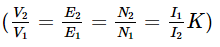Power delivered to load, P = V2 I2
Power transformed = V2 I2 (1 k ) -
power conducted directly = K V2 I2
Ratio of power transformed to total power delivered = (I-k)
Ratio of power conducted directly to power output =K
Where K is transformation ratio:

(Volt ampereratingas an auto transformer / Volt ampereratin as two winding transformer)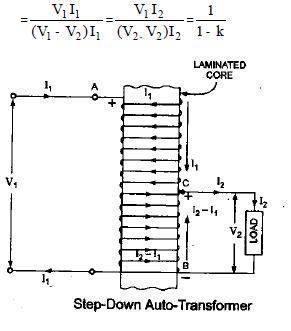(Weight of conductor in an auto transformer / Weight of conductor in a two winding transformer)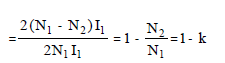Hence saving in conductor material required for winding affected by using an auto- transformer = K× weight of conductor in a two winding transformer.

It provides continuously varying voltage and there is a lot of saving in copper requirements. Weight of copper required on an auto- transformer is (1-K) times of that required in an ordinary two-winding transformer.

Practical Applications. It is used as a balance coil in order to provide a neutral in a 3-Wire ac distribution system, as an auto starter for starting induction and synchronous motors, in electrical testing laboratories, as a regulation transformer and as a booster to raise the voltage in an ac feeder. It is not used in distribution because of low transformation ratio.

Transformer Ratings

A transformer is specified by its kVA (or MVA), voltage and frequency. The kVA rating is the kVa output which the transformer can deliver at rated voltage and frequency under usual operating conditions without exceeding the standard limits of temperature rise.

The document Chapter 3 - AC Machine - Notes, Electrical Machines, Electrical Engineering Notes - Electrical Engineering (EE) is a part of Electrical Engineering (EE) category.
All you need of Electrical Engineering (EE) at this link: Electrical Engineering (EE)Use Code STAYHOME200 and get INR 200 additional OFF

Track your progress, build streaks, highlight & save important lessons and more!

,

,

,

,

,

,

,

,

,

,

,

,

,

,

,

,

,

,

,

,

,

,

,

,

,

,

,

;